# 7. Plotting brain images#

In this section, we detail the general tools to visualize neuroimaging volumes and surfaces with nilearn.

Nilearn comes with plotting function to display brain maps coming from Nifti-like images, in the `nilearn.plotting` module.

## 7.1. Different plotting functions#

Nilearn has a set of plotting functions to plot brain volumes that are fined tuned to specific applications. Amongst other things, they use different heuristics to find cutting coordinates.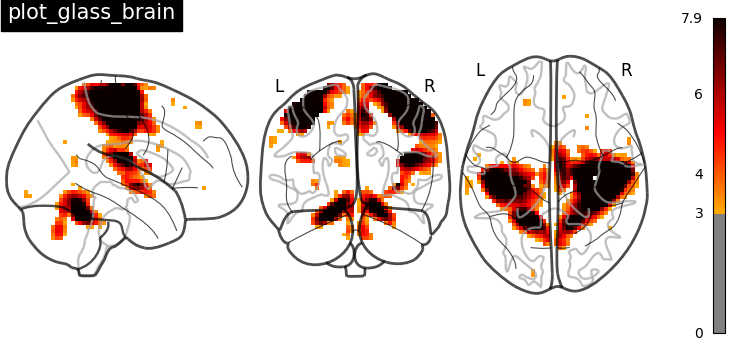`plot_anat`
Plotting an anatomical image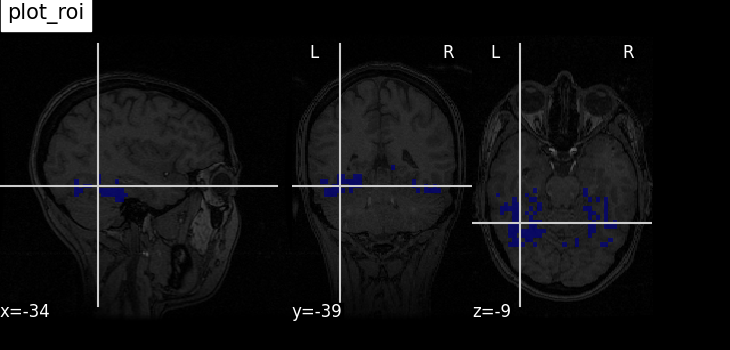`plot_epi`
Plotting an EPI, or T2* image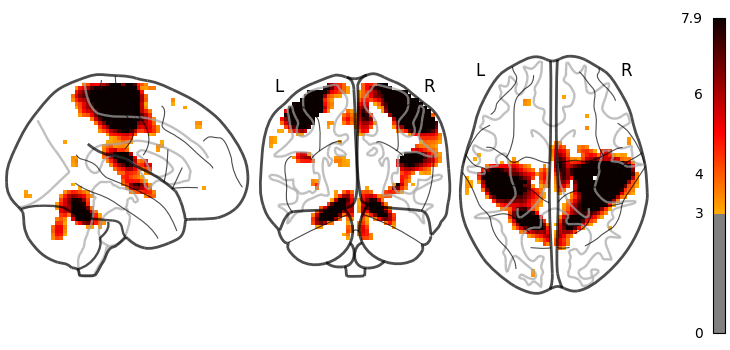`plot_glass_brain`
Glass brain visualization. By default plots maximum intensity projection of the absolute values. To plot positive and negative values set plot_abs parameter to False.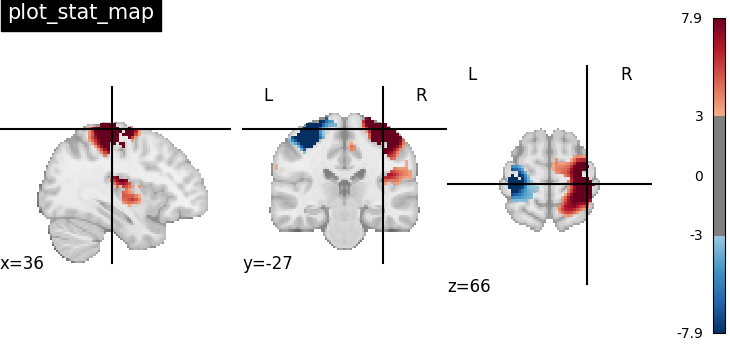`plot_stat_map`
Plotting a statistical map, like a T-map, a Z-map, or an ICA, with an optional background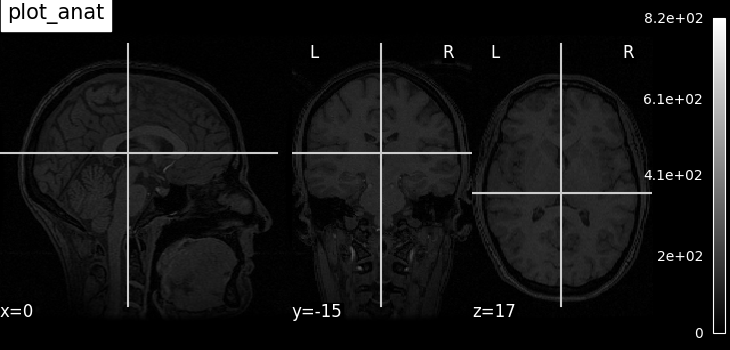`plot_roi`
Plotting ROIs, or a mask, with an optional background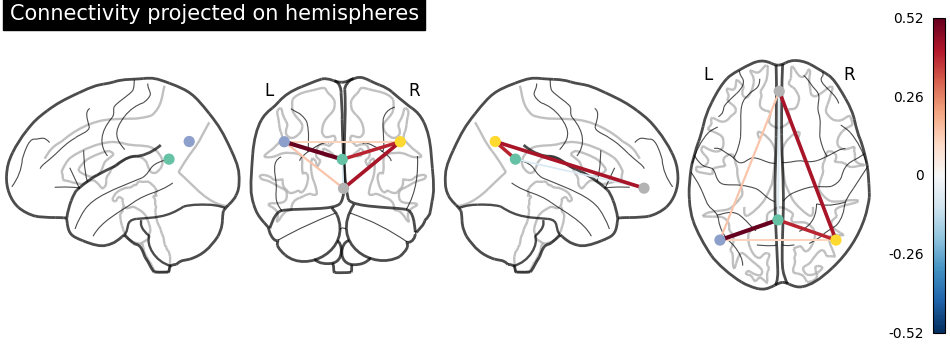`plot_connectome`
Plotting a connectome

Functions for automatic extraction of coords based on brain parcellations useful for `plot_connectome` are demonstrated in Example: Comparing connectomes on different reference atlases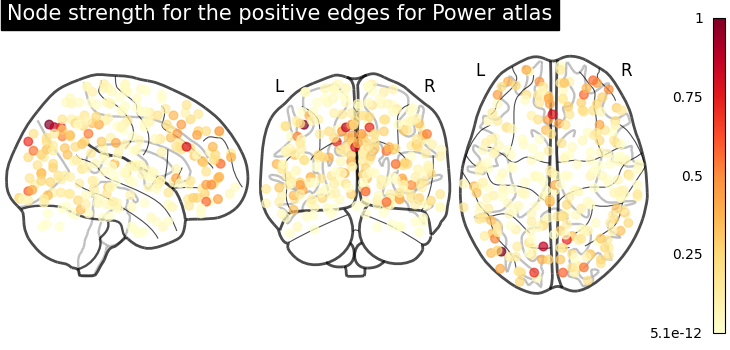`plot_markers`
Plotting network nodes (markers)

Function for automatic plotting of network nodes (markers) and color coding them according to provided nodal measure (i.e. connection strength) as demonstrated in Example: Comparing connectomes on different reference atlases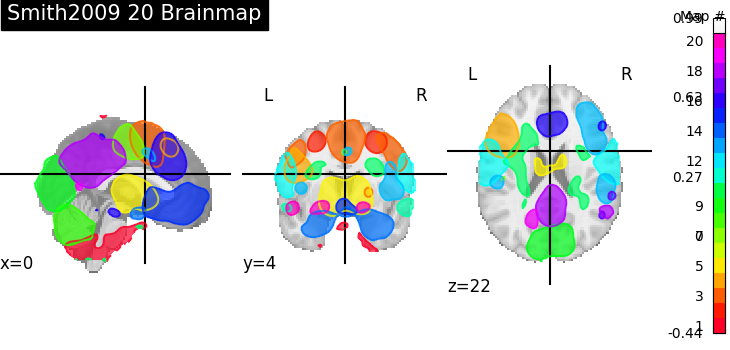`plot_prob_atlas`
Plotting 4D probabilistic atlas maps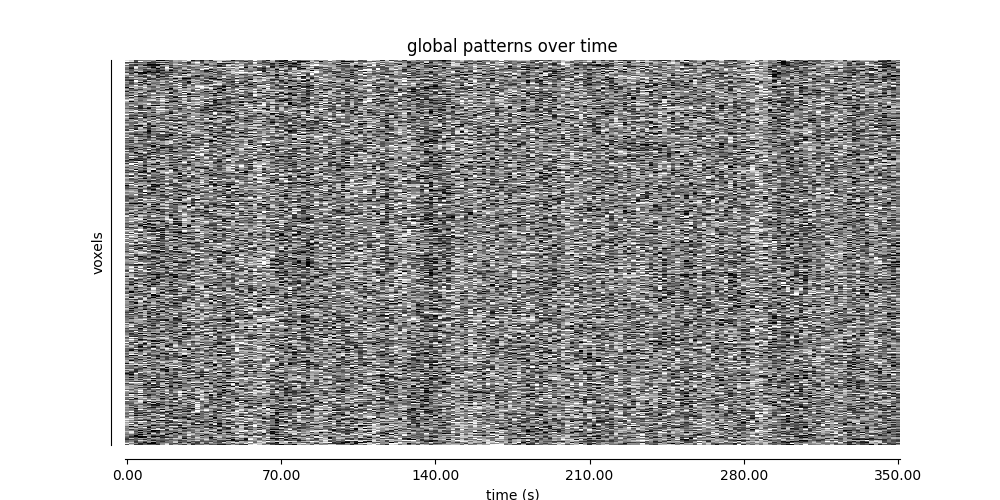`plot_carpet`
Plotting voxel intensities across time.

plot_img

`plot_img`
General-purpose function, with no specific presets

Warning

Opening too many figures without closing

Each call to a plotting function creates a new figure by default. When used in non-interactive settings, such as a script or a program, these are not displayed, but still accumulate and eventually lead to slowing the execution and running out of memory.

To avoid this, you must close the plot as follow:

```from nilearn import plotting
display = plotting.plot_stat_map(img)
display.close()
```

## 7.2. Different display modes#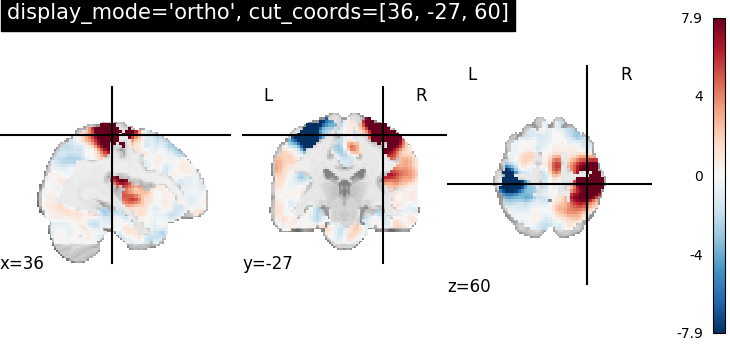`display_mode="ortho", cut_coords=[36, -27, 60]`
Ortho slicer: 3 cuts along the x, y, z directions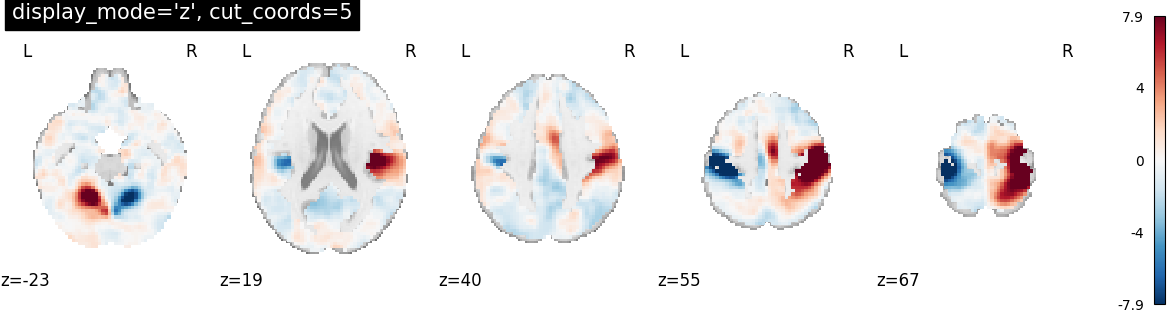`display_mode="z", cut_coords=5`
Cutting in the z direction, specifying the number of cuts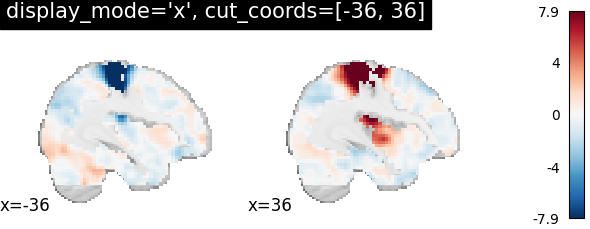`display_mode="x", cut_coords=[-36, 36]`
Cutting in the x direction, specifying the exact cuts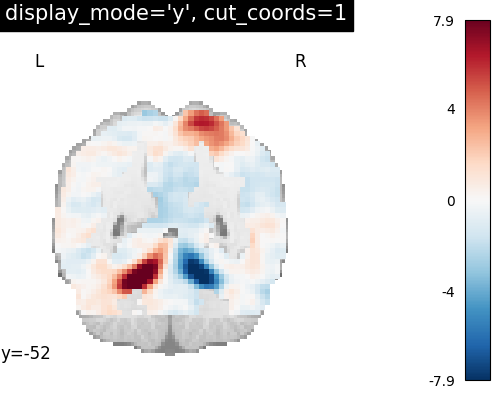`display_mode="y", cut_coords=1`
Cutting in the y direction, with only 1 cut, that is automatically positioned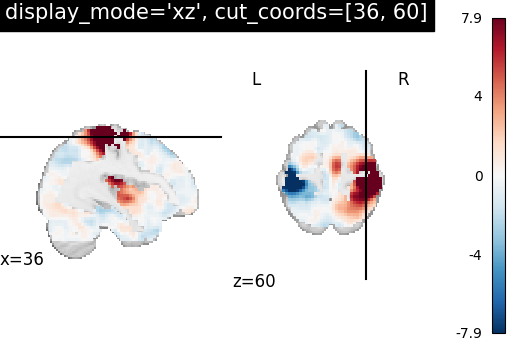`display_mode="z", cut_coords=1, colorbar=False`
Cutting in the z direction, with only 1 cut, that is automatically positioned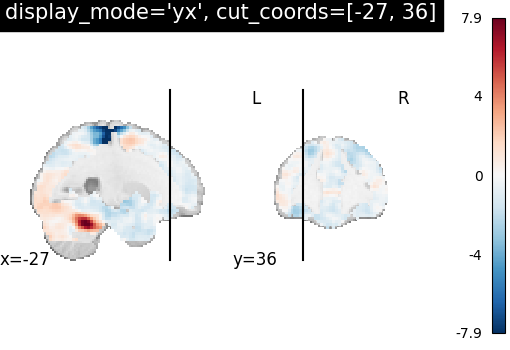`display_mode="xz", cut_coords=[36, 60]`
Cutting in the x and z direction, with cuts manually positioned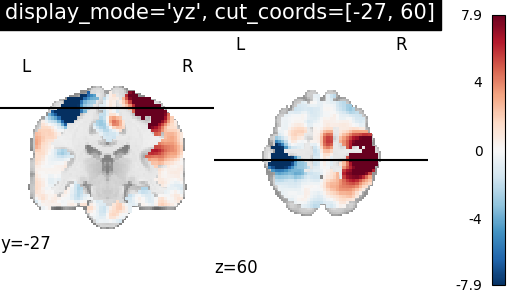`display_mode="yx", cut_coords=[-27, 36]`
Cutting in the y and x direction, with cuts manually positioned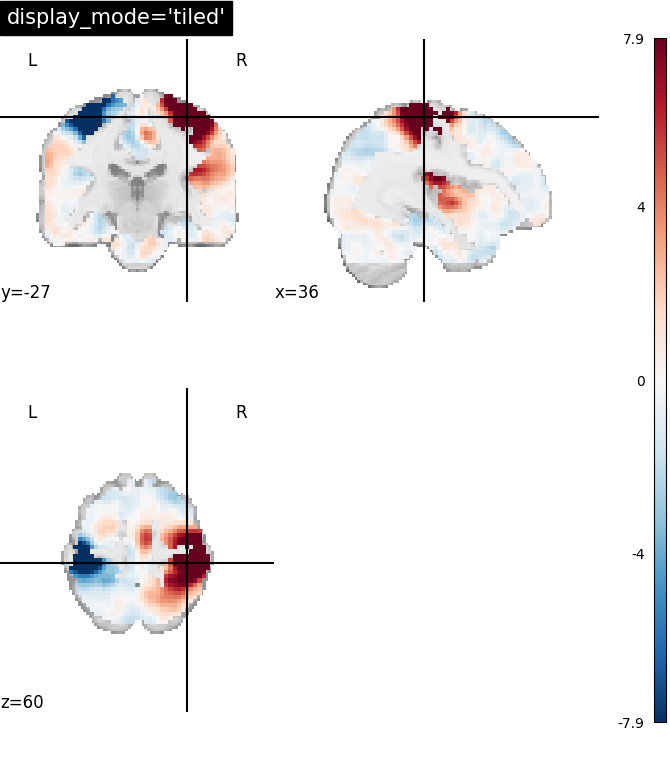`display_mode="yz", cut_coords=[-27, 60]`
Cutting in the y and z direction, with cuts manually positioned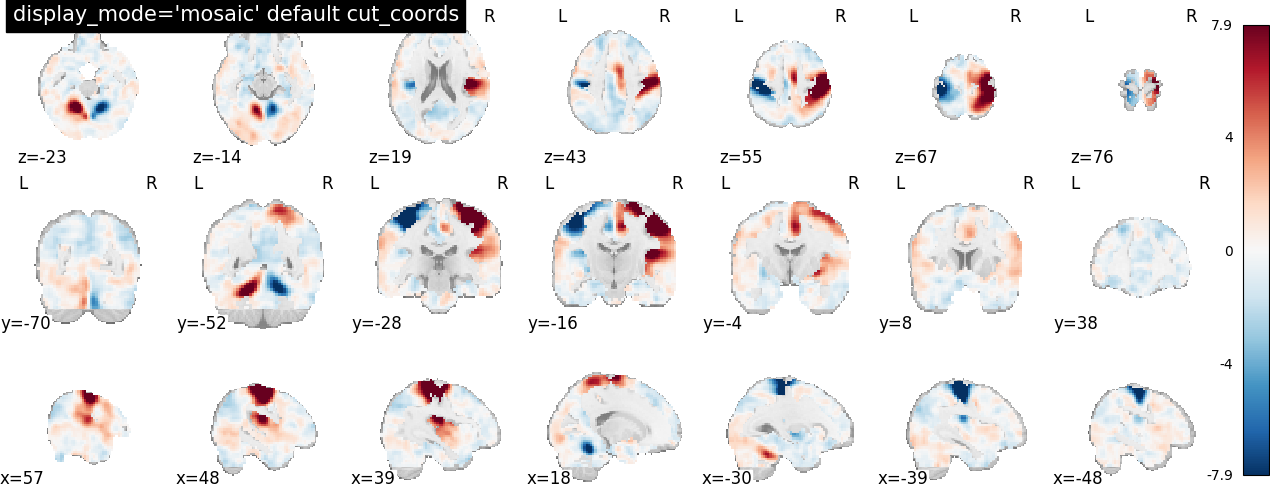`display_mode="tiled", cut_coords=[36, -27, 60]`
Tiled slicer: 3 cuts along the x, y, z directions, arranged in a 2x2 grid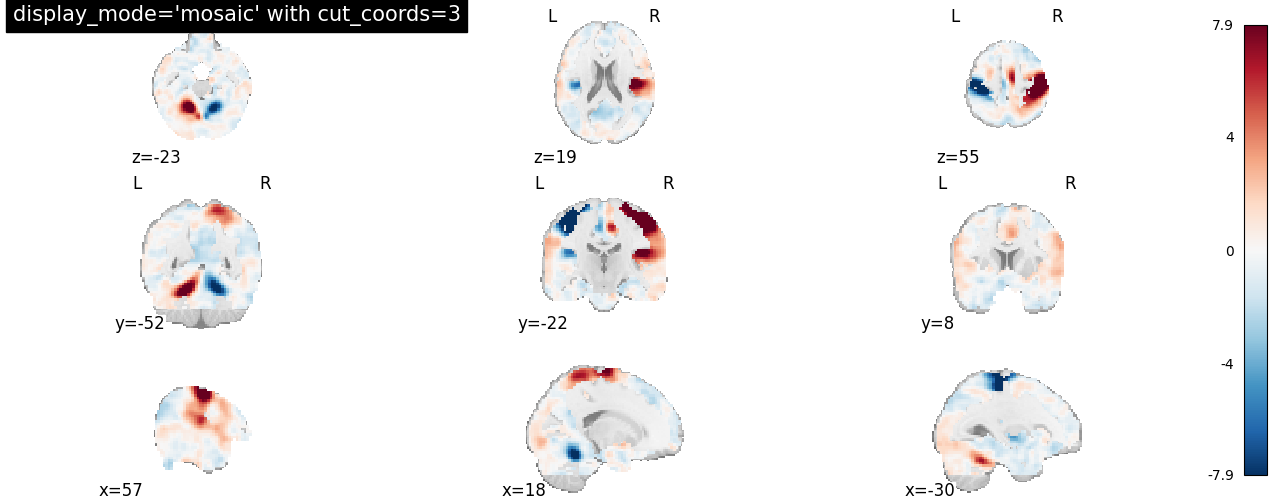`display_mode="mosaic"`
Mosaic slicer: multiple cuts along the x, y, z directions, with cuts automatically positioned by default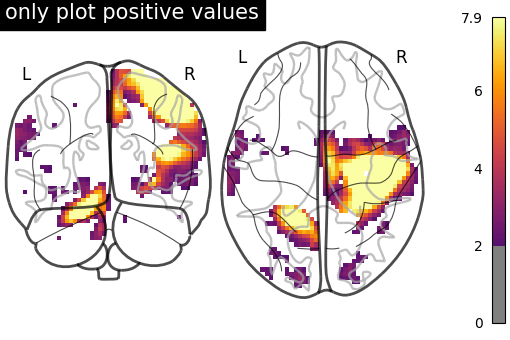`Glass brain display_mode="lzr"`
Glass brain and Connectome provide additional display modes due to the possibility of doing hemispheric projections. Check out: `'l'`, `'r'`, `'lr'`, `'lzr'`, `'lyr'`, ‘`lzry'`, `'lyrz'`.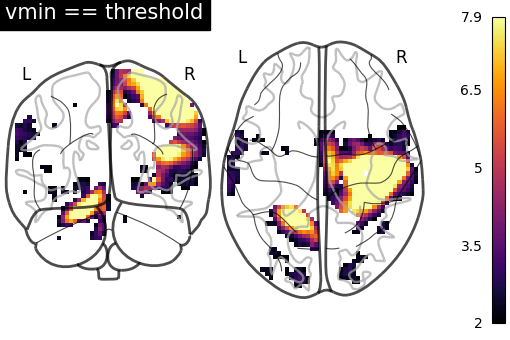`Glass brain display_mode="lyrz"`
Glass brain and Connectome provide additional display modes due to the possibility of doing hemispheric projections. Check out: `'l'`, `'r'`, `'lr'`, `'lzr'`, `'lyr'`, ‘`lzry'`, `'lyrz'`.

## 7.3. Available Colormaps#

Nilearn plotting library ships with a set of extra colormaps, as seen in the image below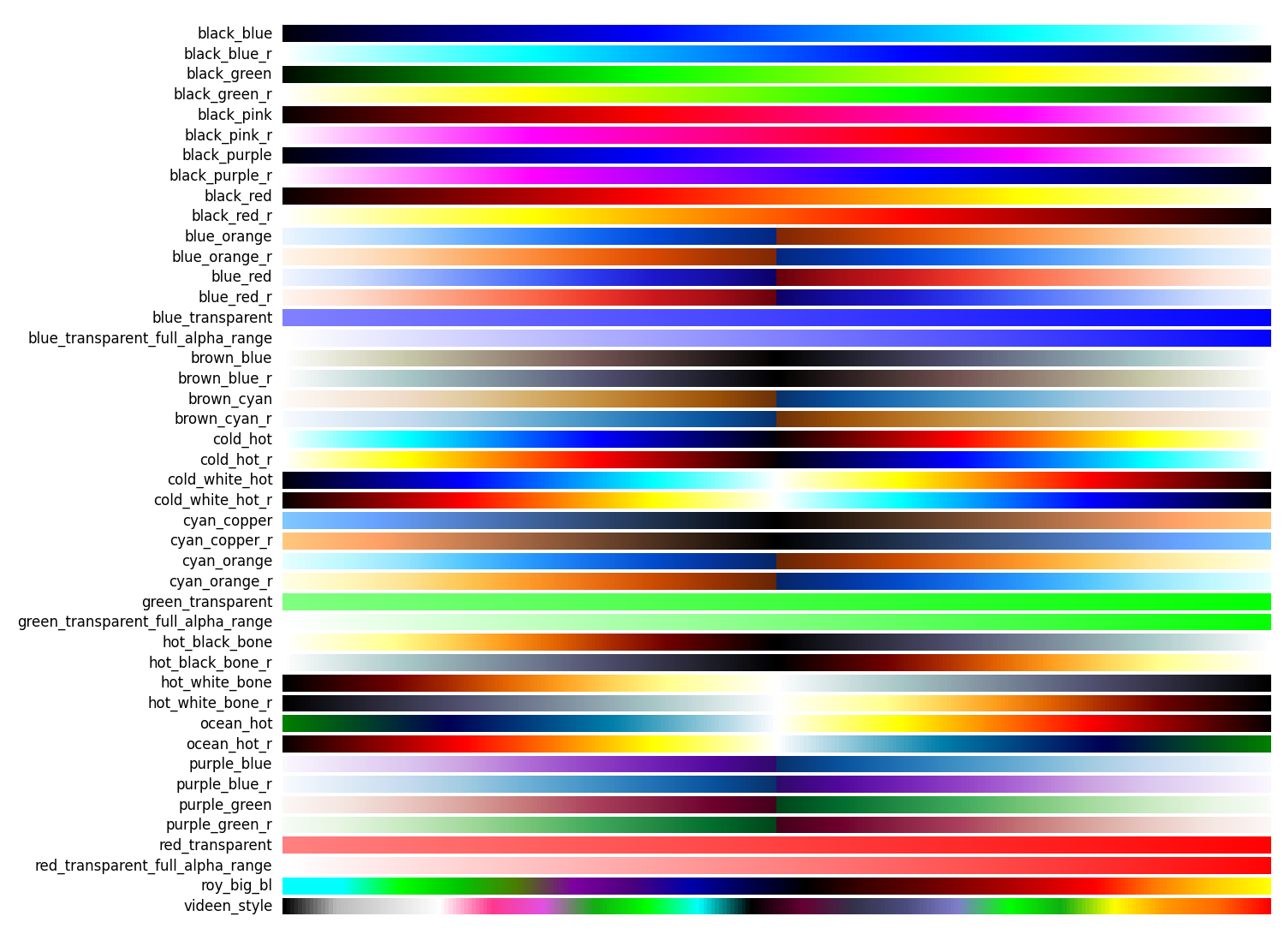These colormaps can be used as any other matplotlib colormap.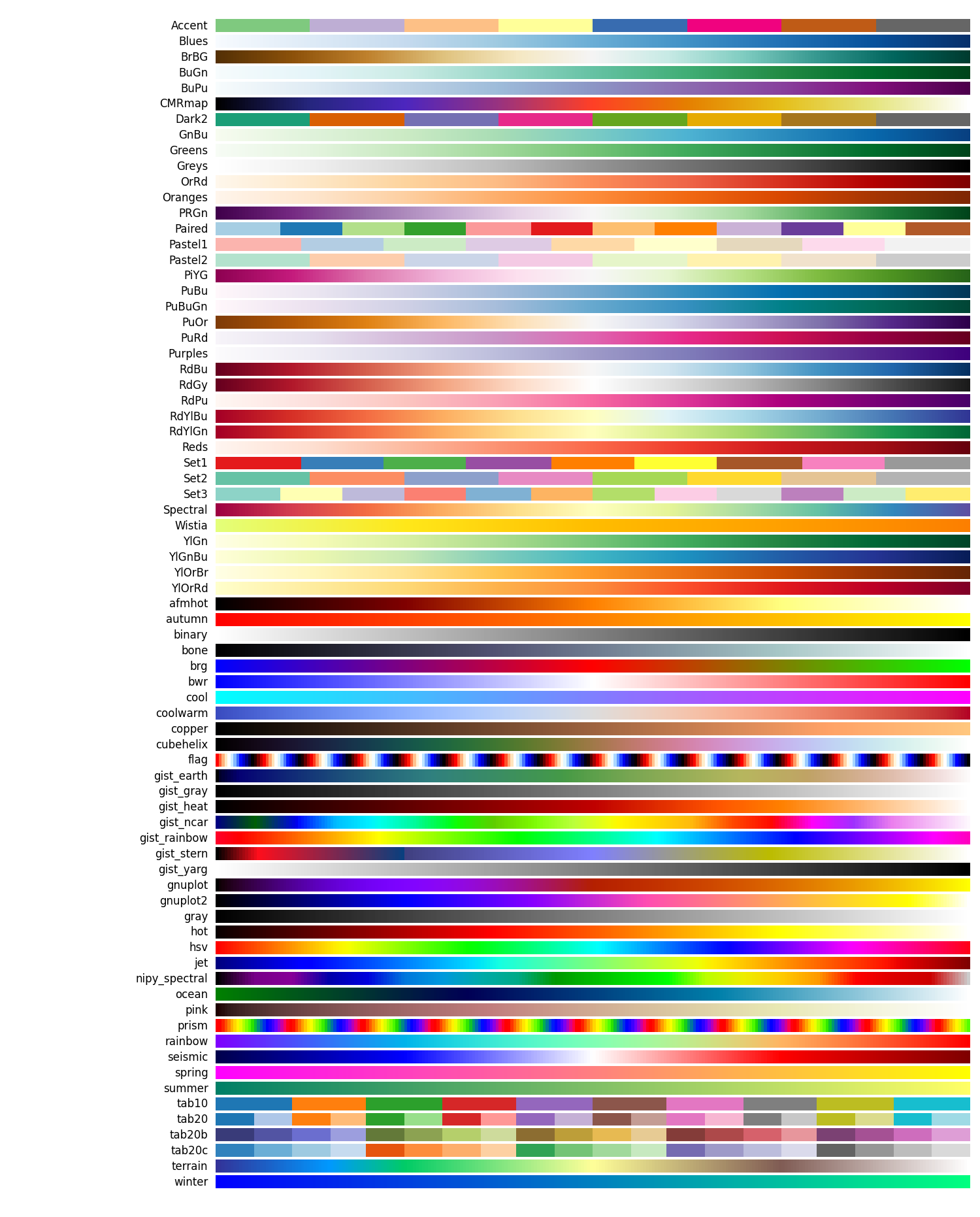## 7.4. Adding overlays, edges, contours, contour fillings, markers, scale bar#

To add overlays, contours, or edges, use the return value of the plotting functions. Indeed, these return a display object, such as the `nilearn.plotting.displays.OrthoSlicer`. This object represents the plot, and has methods to add overlays, contours or edge maps:

```display = plotting.plot_epi(...)
```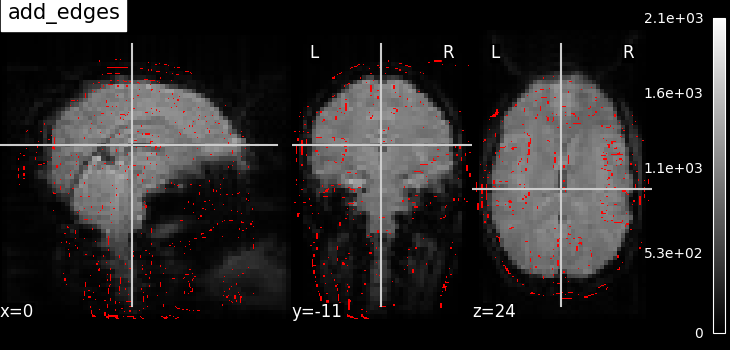`display.add_edges(img)`
Add a plot of the edges of `img`, where edges are extracted using a Canny edge-detection routine. This is typically useful to check registration. Note that `img` should have some visible sharp edges. Typically an EPI img does not, but a T1 does.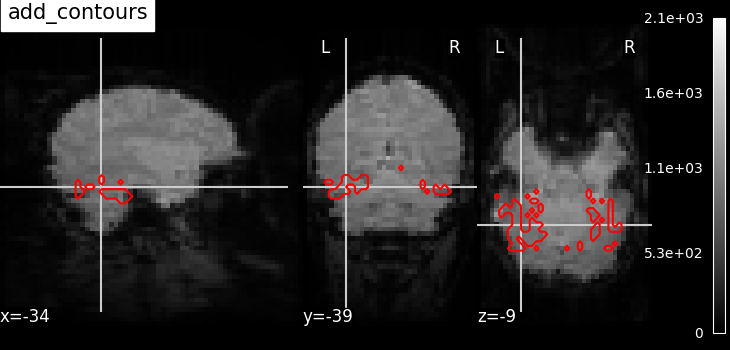`display.add_contours(img, levels=[0.5], colors="r")`
Add a plot of the contours of `img`, where contours are computed for constant values, specified in ‘levels’. This is typically useful to outline a mask, or ROI on top of another map.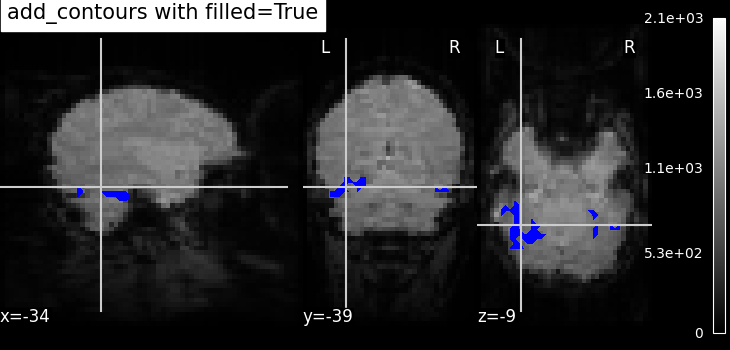`display.add_contours(img, filled=True, alpha=0.7, levels=[0.5], colors="b")`
Add a plot of `img` with contours filled with colors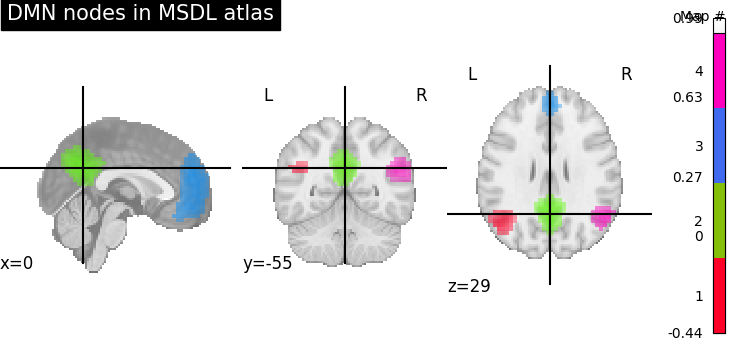`display.add_overlay(img, cmap=plotting.cm.purple_green, threshold=3)`
Add a new overlay on the existing figure
Example: Visualizing a probabilistic atlas: the default mode in the MSDL atlas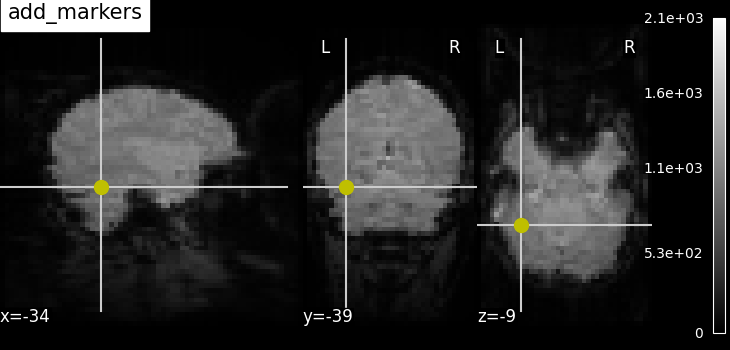`display.add_markers(coords, marker_color="y", marker_size=100)`
Add seed based MNI coordinates as spheres on top of statistical image or EPI image. This is useful for seed based regions specific interpretation of brain images.
Example: Producing single subject maps of seed-to-voxel correlation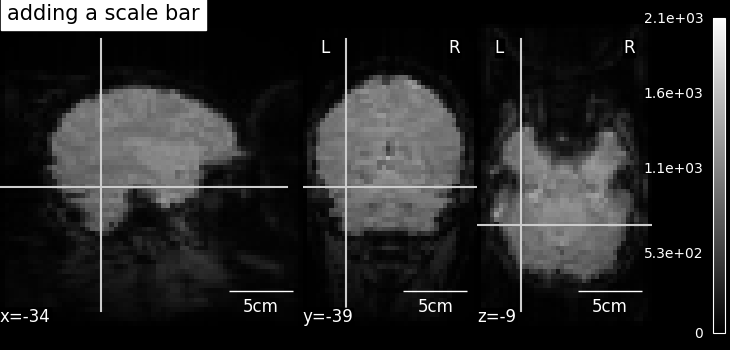`display.annotate(scalebar=True)`
Adds annotations such as a scale bar, or the cross of the cut coordinates
Example: More plotting tools from nilearn

## 7.5. Displaying or saving to an image file#

To display the figure when running a script, you need to call `nilearn.plotting.show` (this is just an alias to `matplotlib.pyplot.show`):

```from nilearn import plotting
plotting.show()
```

The simplest way to output an image file from the plotting functions is to specify the `output_file` argument:

```from nilearn import plotting
plotting.plot_stat_map(img, output_file='pretty_brain.png')
```

In this case, the display is closed automatically and the plotting function returns None.

The display object returned by the plotting function has a savefig method that can be used to save the plot to an image file:

```from nilearn import plotting
display = plotting.plot_stat_map(img)
display.savefig('pretty_brain.png')
# Remember to close the display
display.close()
```

## 7.6. Surface plotting#

Plotting functions required to plot surface data or statistical maps on a brain surface.

New in version 0.3.

## 7.7. Interactive plots#

Nilearn also has functions for making interactive plots that can be seen in a web browser. There are two kinds of plots for which an interactive mode is available:

New in version 0.5: Interactive plotting is new in nilearn 0.5

New in version 0.9.0: Nilearn offers the possibility to select different plotting engines (either matplotlib or plotly) for most surface plotting functions.

### 7.7.1. 3D Plots of statistical maps or atlases on the cortical surface#

For 3D surface plots of statistical maps or surface atlases, you have different options depending on what you want to do and the packages you have installed.

• If you have plotly installed: Since Nilearn `0.9.0`, it is possible to use plotly as the plotting engine in most surface plotting functions of Nilearn like `plot_surf`, `plot_surf_stat_map`, or `plot_surf_roi`. By default these functions use matplotlib as the plotting engine which results in non-interactive plots. By setting `engine='plotly'`, an interactive version of these plots will be automatically opened in your web browser. In addition, if you have kaleido installed, you can save the plots automatically to png files. Finally, the plotly figure returned can be further customized in order to make more elaborate plots.

• If you don’t have plotly:

#### 7.7.1.1. `view_img_on_surf`: Surface plot using a 3D statistical map#

You can use `view_img_on_surf` to display a 3D statistical map projected on the cortical surface:

```from nilearn import plotting, datasets
view = plotting.view_img_on_surf(img, threshold='90%', surf_mesh='fsaverage')
```

If you are running a notebook, displaying `view` will embed an interactive plot (this is the case for all interactive plots produced by nilearn’s “view” functions):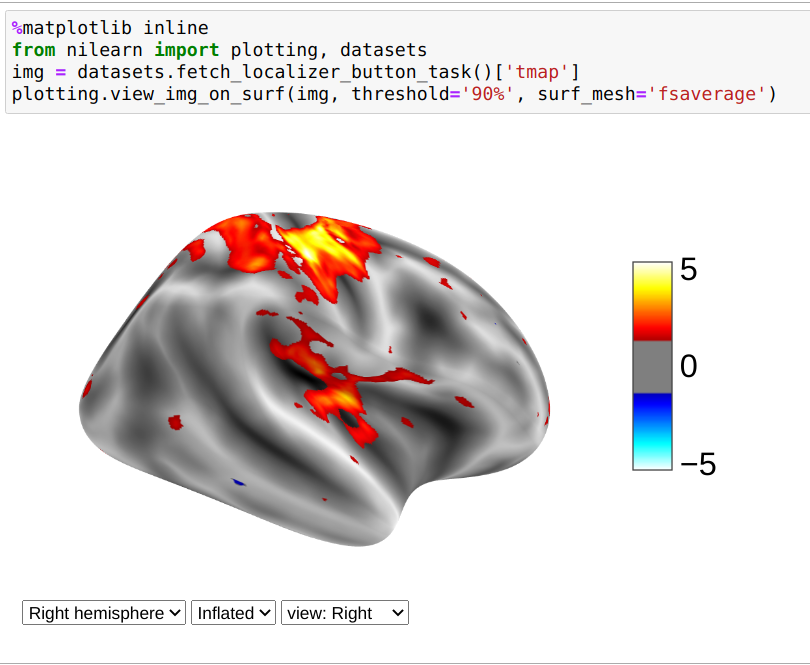If you are not using a notebook, you can open the plot in a browser like this:

```view.open_in_browser()
```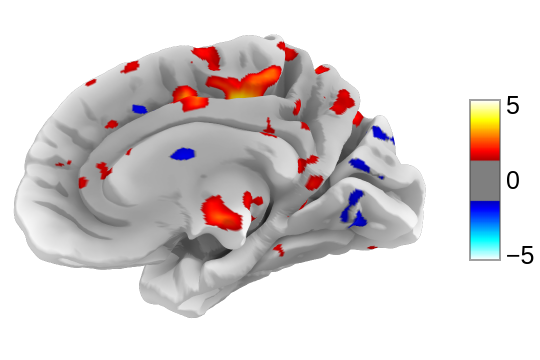Or you can save it to an html file:

```view.save_as_html("surface_plot.html")
```

#### 7.7.1.2. `view_surf`: Surface plot using a surface map and a cortical mesh#

You can use `view_surf` to display a 3D surface statistical map over a cortical mesh:

```from nilearn import plotting, datasets
destrieux = datasets.fetch_atlas_surf_destrieux()
fsaverage = datasets.fetch_surf_fsaverage()
view = plotting.view_surf(fsaverage['infl_left'], destrieux['map_left'],
cmap='gist_ncar', symmetric_cmap=False)
view.open_in_browser()
```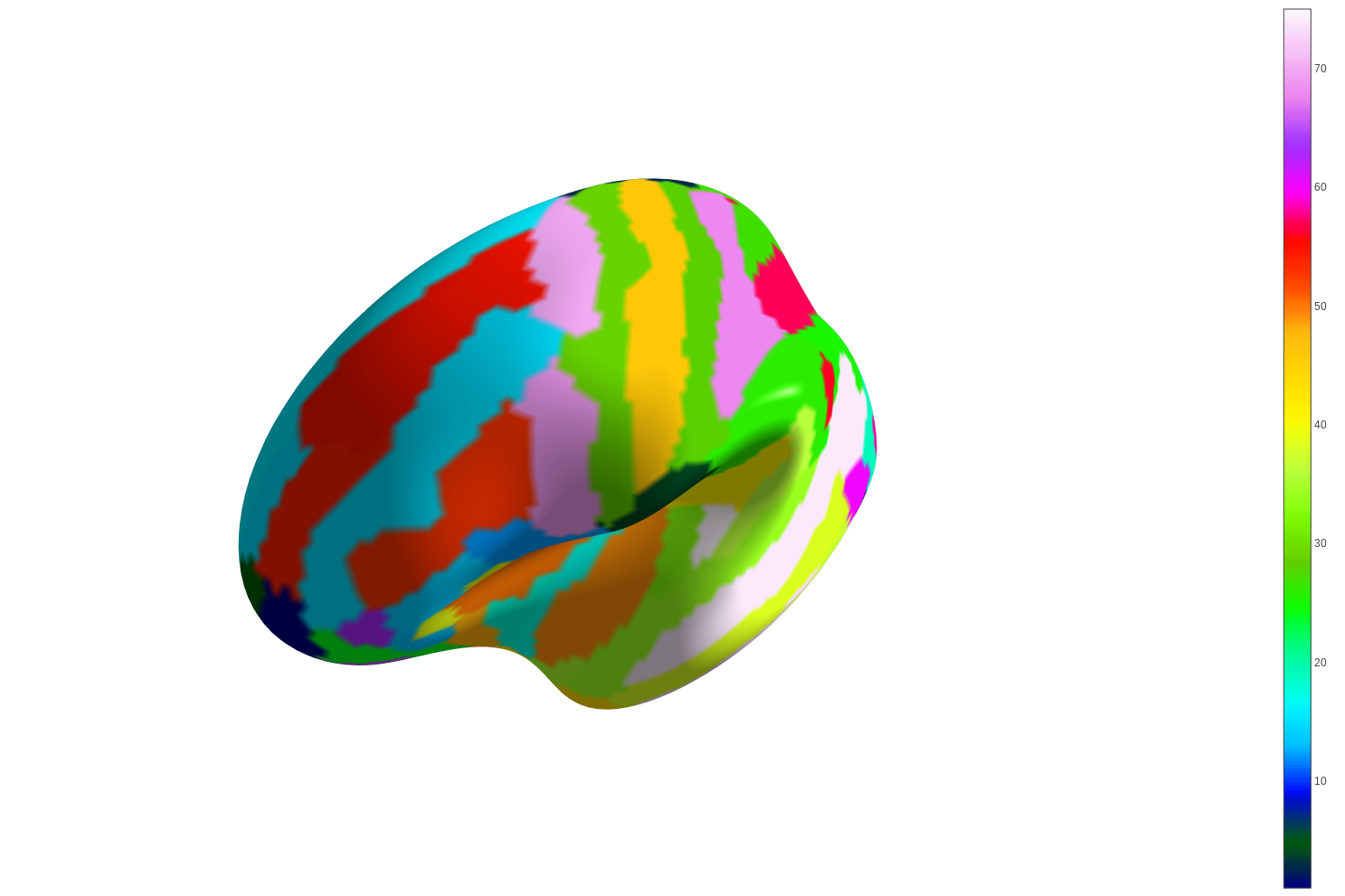#### 7.7.1.3. `plot_surf_stat_map`: Surface plot using a surface map and a cortical mesh#

If you have plotly installed, you can also use `plot_surf_stat_map` with the `engine` parameter set to “plotly” to display a statistical map over a cortical mesh:

```from nilearn import plotting, datasets, surface
fsaverage = datasets.fetch_surf_fsaverage()
map = surface.vol_to_surf(motor_images.images, mesh)
fig = plotting.plot_surf_stat_map(mesh, map, hemi='right',
view='lateral', colorbar=True,
threshold=1.2,
bg_map=fsaverage.sulc_right,
engine='plotly')
fig.show()
```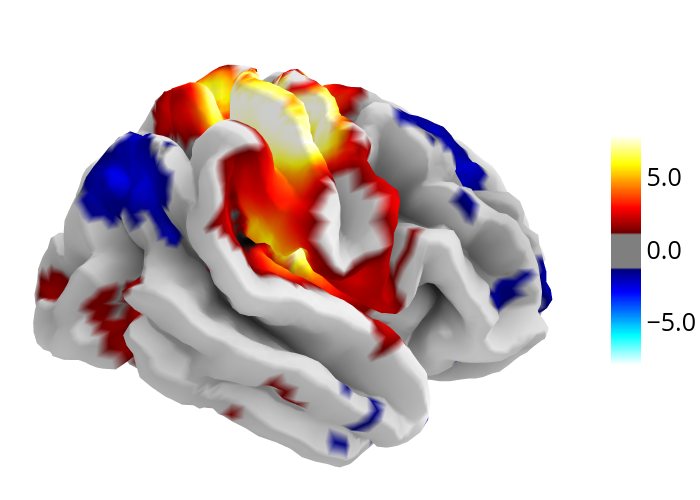### 7.7.2. 3D Plots of connectomes#

For 3D plots of a connectome, use `view_connectome`. To see only markers, use `view_markers`.

`view_connectome`: 3D plot of a connectome:

```view = plotting.view_connectome(correlation_matrix, coords, edge_threshold='90%')
view.open_in_browser()
```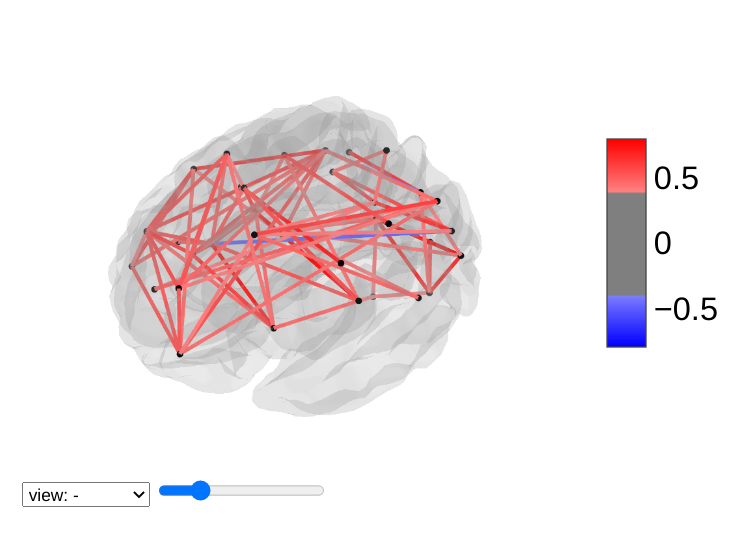### 7.7.3. 3D Plots of markers#

`view_markers`: showing markers (e.g. seed locations) in 3D:

```from nilearn import plotting
dmn_coords = [(0, -52, 18), (-46, -68, 32), (46, -68, 32), (1, 50, -5)]
view = plotting.view_markers(dmn_coords, ['red', 'cyan', 'magenta', 'orange'],
marker_size=10)
view.open_in_browser()
```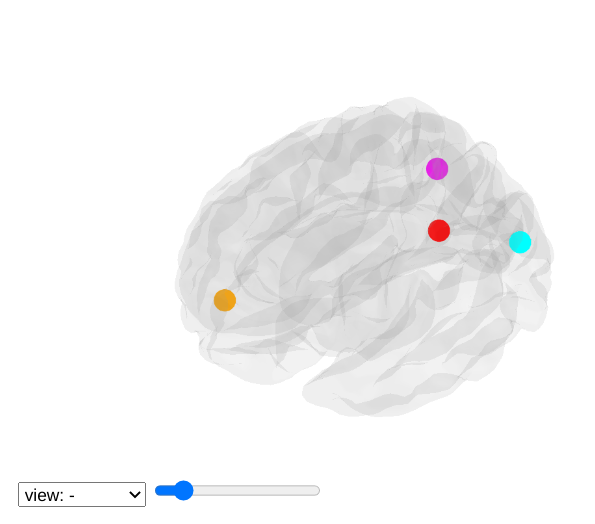### 7.7.4. Interactive visualization of statistical map slices#

`view_img`: open stat map in a Brainsprite viewer (https://github.com/simexp/brainsprite.js):

```from nilearn import plotting, datasets
html_view = plotting.view_img(img, threshold=2, vmax=4,
cut_coords=[-42, -16, 52],
title="Motor contrast")
```

in a Jupyter notebook, if `html_view` is not requested, the viewer will be inserted in the notebook: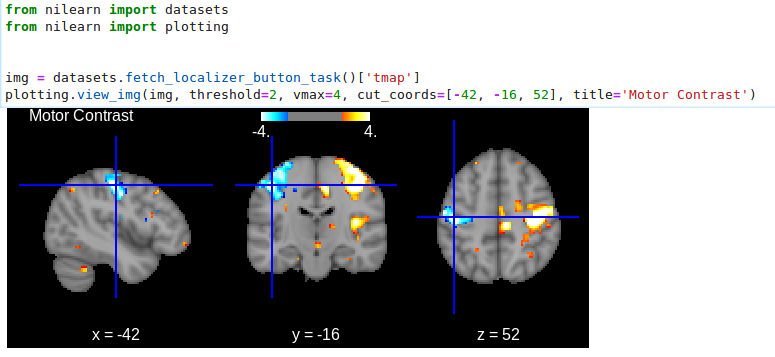```html_view.open_in_browser()
```html_view.save_as_html('viewer.html')